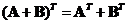## Matrix Addition

When we have two matrices,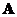and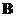then the sum of the two matrices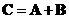is a matrix whose entry is equal to the sum of the corresponding entries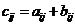.
Matrix addition works only when the two matrices have the same size and the result is also the same size as the input matrices.

Example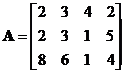,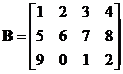,The interactive program below shows you the result of matrix addition. Your input must be two matrices of the same size (one matrix for each text box). Random Example will generate random matrices of the same size.

+

## Properties

Some important properties of matrix addition are

• Matrix addition is a commutative operation, that you can reverse the order and still get the same result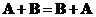• Matrix addition is an associative operation, that is exchanging the parentheses (to say which order to be computed first) does not change the result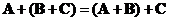.
• Null matrix (or zero matrix) is a unique additive identity matrix such that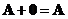. The null matrix has the same size as matrix.
• Transpose of the matrix addition is equal to the addition of their transposes